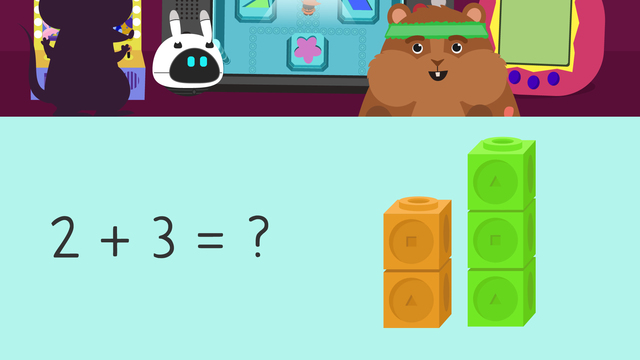Rating

Ø 5.0 / 1 ratings
The authorsTeam Digital
CCSS.MATH.CONTENT.K.OA.A.1

## Basics on the topicAdding with Objects

Adding means putting two or more numbers together to make a larger amount. When we adding objects, we use things we can touch to show us the numbers we are putting together. These help us count out the new amount. In math, we can use lots of objects to help us show numbers, like counters, place value blocks, and math cubes.

## Adding with Objects – Example

Let’s use math cubes with the example two plus three. These objects will help! To start, we will use math cubes to show the first number, two.Then, we will use math cubes to show the second number, three.Next, bring the two cubes and the three cubes together into one, bigger group, and count them all!Five is the answer so we write it after the equal sign.## Adding with Objects – Summary of Steps

Using objects such as math cubes may help you to depict addition in math. If you want to use math cubes for addition, these are the steps to follow:

Step # What to do
1 Use math cubes to represent
the first and the second number.
2 Bring both sets of cubes
together and count them all!
the equal sign.
• Use math cubes to show the first number.

• Then, use math cubes to show the second number.

• Next, bring both sets of cubes together and count them all!